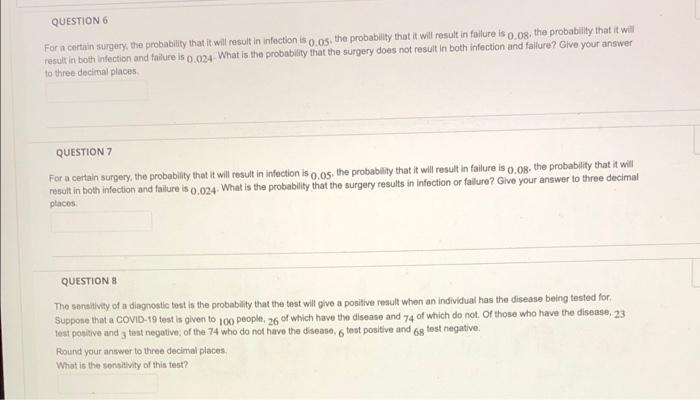# Question Solved1 Answer QUESTION 6 For a certain surgery, the probability that it will result in infection is 0.05, the probability that it will result in failure is 0.08. the probability that it will result in both infection and failure is 0.024- What is the probability that the surgery does not result in both infection and failure? Give your answer to three decimal places. QUESTION 6 For a certain surgery, the probability that it will result in infection is 0.05, the probability that it will result in failure is 0.08. the probability that it will result in both infection and failure is 0.024- What is the probability that the surgery does not result in both infection and failure? Give your answer to three decimal places. QUESTION 7 For a certain surgery, the probability that it will result in infection is 0.05. the probability that it will result in failure is 0.08. the probability that it will result in both infection and failure is 0.024. What is the probability that the surgery results in infection or failure? Give your answer to three decimal places. QUESTION 8 The sensitivity of a diagnostic test is the probability that the test will give a positive result when an individual has the disease being tested for. Suppose that a COVID-19 test is given to 100 people, 26 of which have the disease and 74 of which do not. Of those who have the disease, 23 test positive and 3 test negative; of the 74 who do not have the disease, 6 test positive and 68 test negative. Round your answer to three decimal places. What is the sensitivity of this test?DPH8Z5 The Asker · Probability and StatisticsTranscribed Image Text: QUESTION 6 For a certain surgery, the probability that it will result in infection is 0.05, the probability that it will result in failure is 0.08. the probability that it will result in both infection and failure is 0.024- What is the probability that the surgery does not result in both infection and failure? Give your answer to three decimal places. QUESTION 7 For a certain surgery, the probability that it will result in infection is 0.05. the probability that it will result in failure is 0.08. the probability that it will result in both infection and failure is 0.024. What is the probability that the surgery results in infection or failure? Give your answer to three decimal places. QUESTION 8 The sensitivity of a diagnostic test is the probability that the test will give a positive result when an individual has the disease being tested for. Suppose that a COVID-19 test is given to 100 people, 26 of which have the disease and 74 of which do not. Of those who have the disease, 23 test positive and 3 test negative; of the 74 who do not have the disease, 6 test positive and 68 test negative. Round your answer to three decimal places. What is the sensitivity of this test?
More
Transcribed Image Text: QUESTION 6 For a certain surgery, the probability that it will result in infection is 0.05, the probability that it will result in failure is 0.08. the probability that it will result in both infection and failure is 0.024- What is the probability that the surgery does not result in both infection and failure? Give your answer to three decimal places. QUESTION 7 For a certain surgery, the probability that it will result in infection is 0.05. the probability that it will result in failure is 0.08. the probability that it will result in both infection and failure is 0.024. What is the probability that the surgery results in infection or failure? Give your answer to three decimal places. QUESTION 8 The sensitivity of a diagnostic test is the probability that the test will give a positive result when an individual has the disease being tested for. Suppose that a COVID-19 test is given to 100 people, 26 of which have the disease and 74 of which do not. Of those who have the disease, 23 test positive and 3 test negative; of the 74 who do not have the disease, 6 test positive and 68 test negative. Round your answer to three decimal places. What is the sensitivity of this test?